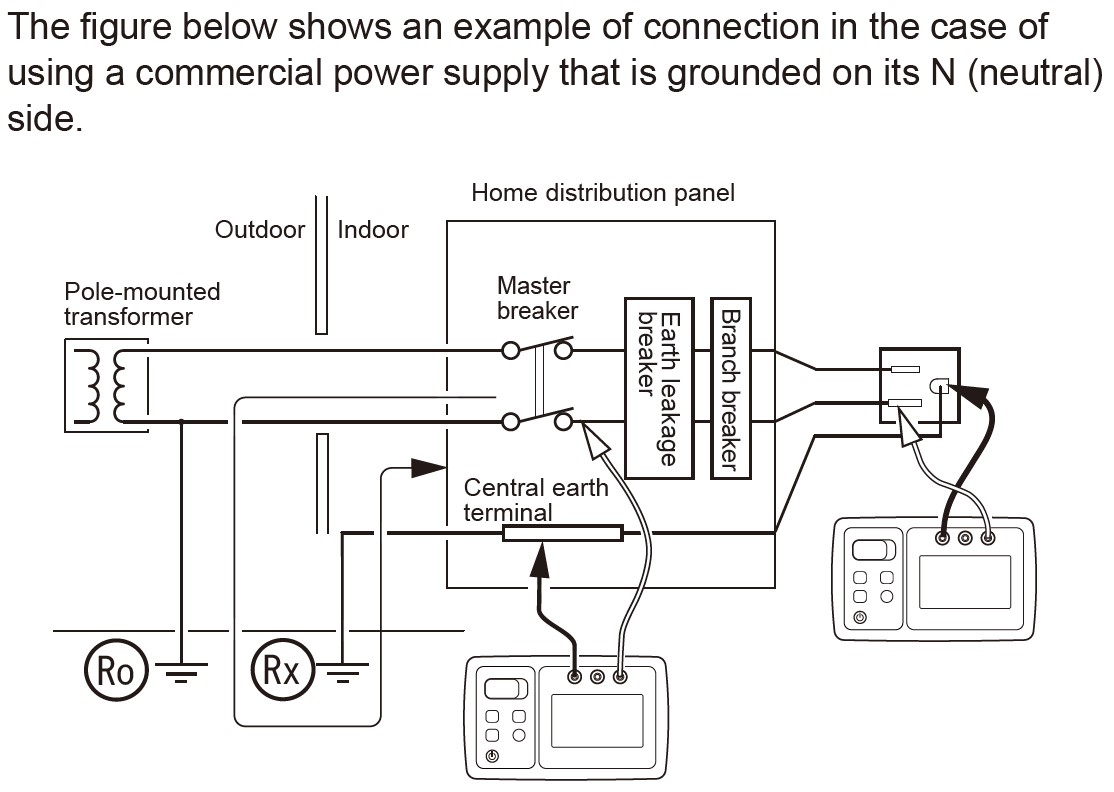# What is two-point (two-pole) method of Earth Tester?

## QWhat is the 2-point (2-pole) measurement method of Earth Tester FT6031-50?

A

The simplified measurement method (2-point or 2-pole method) checks the "grounding resistance of equipment grounding" of the earthing system called the TT method.
When an auxiliary ground rod cannot be inserted, use an existing low grounding resistor as an auxiliary electrode to obtain grounding resistance.

Based on the measurement principle, the measurement value of this method is below (also see image):
Sum of grounding resistance value of the "object to be measured" (Rx) and grounding resistance value of the "existing grounding resistor already used" (Ro). -> Rx + Ro

Therefore, the grounding resistance value of the existing grounding resistive element to be used, must be lower than the value of the grounding point to be measured.

In the simplified measurement method, the resistance value of the grounding resistor to be used is added to the measurement result.
Measurement of less than 10 Ω is extremely difficult with this simplified measurement method.

•